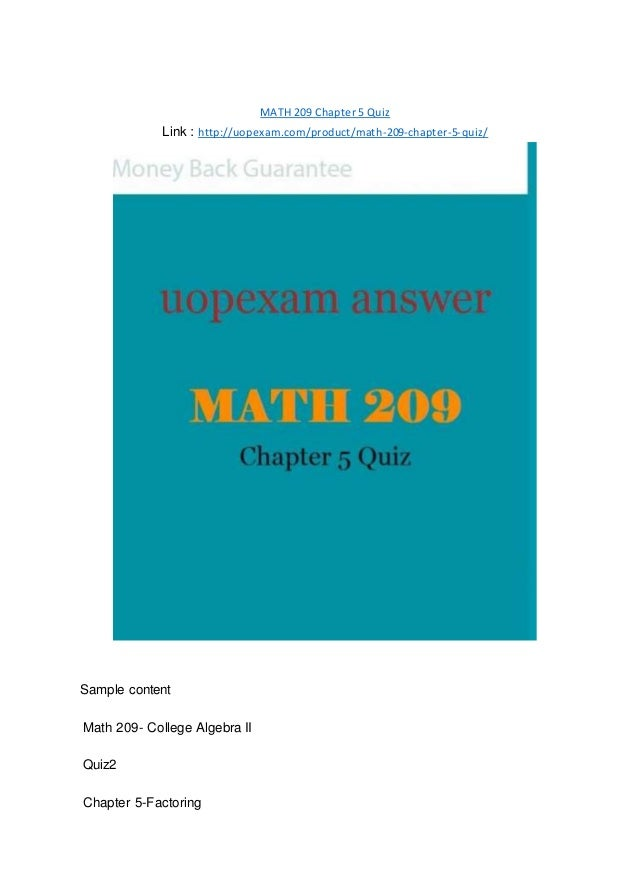Successfully reported this slideshow.Upcoming SlideShare
×

# MATH 209 Chapter 5 Quiz 2015 version

Sample content

Math 209- College Algebra II

Quiz2

Chapter 5-Factoring

50 points

Name:

Work the following problems. Show all your own work for maximum credit. Each problem is worth 5 points. Post your work in your individual forum.

Please put the quiz number and your name in the email subject and put the following information at the top of the assignment.

Name

Course Name

Assignment Title

Date

1. Answer the following question with complete sentences:

➢ What is a prime number?

Any number divisible by itself and 1 only is called a prime number

Exception to this case is 1 which is not a prime number

➢ What does it mean to factor an expression?

Factoring an expression means taking out zeroes from an expression

For example

X2 +5x+6

It can be factored as

(x+3)(x+2)

➢ What is the ac method of factoring? Give an example.

Consider a polynomial expression of the form ax2 + bx + c.

The polynomial can be factored if there ar

• Full Name
Comment goes here.

Are you sure you want to Yes No